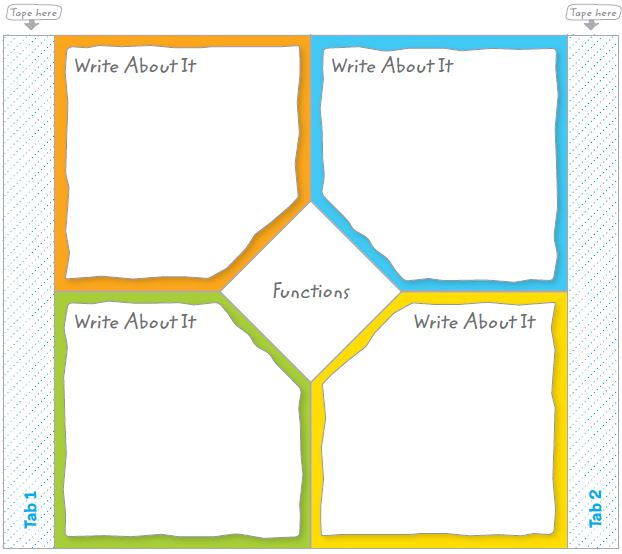Homework Explained - Math Practice 101Dear guest, you are not a registered member. As a guest, you only have read-only access to our books, tests and other practice materials.

As a registered member you can:

Registration is free and doesn't require any type of payment information. Click here to Register.
Go to page:
Chapter 8:Functions and Inequalities; Mid-Chapter Review

### Key Concept Check

• Question 1

Use your Foldable to help review the chapter.### Got it?

Circle the correct term or number to complete each sentence.

• Question 1

The next number in the sequence 12, 15, 18, 21, . . . is (24, 27).

• Question 2

The output of a function is the (independent, dependent) variable.

• Question 3

A(n) (arithmetic, geometric) sequence can be found by multiplying each previous term by the same number.

• Question 4

The input of a function is the (independent, dependent) variable.

• Question 5

A(n)(inequality, function) is a relation that assigns exactly one output value to one input value.

Yes, email page to my online tutor. (if you didn't add a tutor yet, you can add one here)